The Radius of the Midsphere of a Polyhedron, also called the Interradius. For a Regular Polyhedron with Schläfli Symbol, the Dual Polyhedron is. Denote the Inradius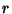, midradius, and Circumradius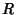, and let the side length be. Then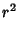(1)(2)

For Regular Polyhedra and Uniform Polyhedra, the Dual Polyhedron has Circumradiusand Inradius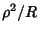. Let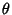be the Angle subtended by the Edge of an Archimedean Solid. Then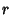(3)(4)(5)

so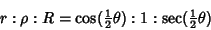(6)

(Cundy and Rollett 1989). Expressing the midradius in terms of the Inradiusand Circumradiusgives(7)

for an Archimedean Solid.

References

Cundy, H. and Rollett, A. Mathematical Models, 3rd ed. Stradbroke, England: Tarquin Pub., pp. 126-127, 1989.